# Diffraction at a single slit a a2 Semi

• Slides: 23
Download presentationDiffraction at a single slit a=λ a=2λ Semi circular wave fronts First minima & maxima become visible a=4λ Diffraction is the spreading of wavefronts around corners and obstacles. If the slit gets narrower diffraction increases. If the wavelength increases diffraction increases.Diffraction at a single slit a=λ a=2λ Semi circular wave fronts First minima & maxima become visible a=4λ Diffraction is the spreading of wavefronts around corners and obstacles. If the slit gets narrower diffraction increases. If the wavelength increases diffraction increases.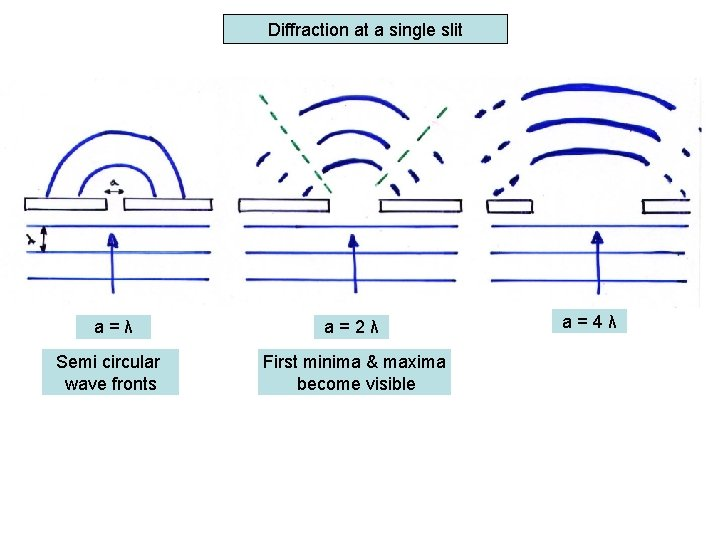Diffraction at a single slit a=λ a=2λ Semi circular wave fronts First minima & maxima become visible a=4λ Diffraction is the spreading of wavefronts around corners and obstacles. If the slit gets narrower diffraction increases. If the wavelength increases diffraction increases.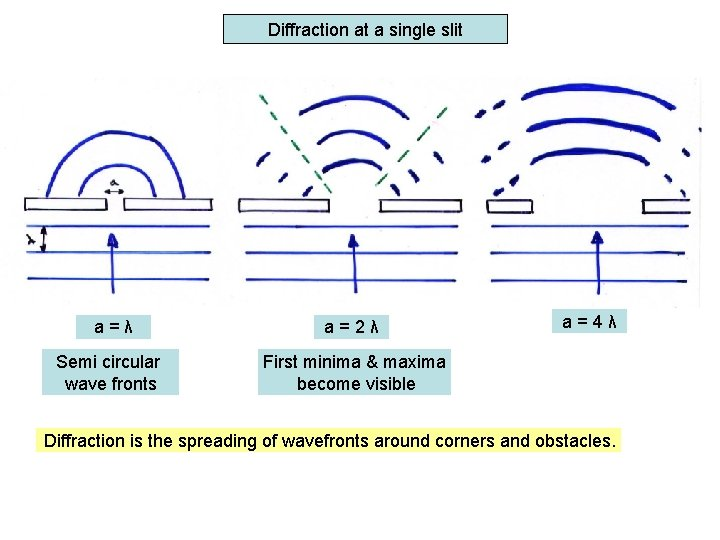Diffraction at a single slit a=λ a=2λ Semi circular wave fronts First minima & maxima become visible a=4λ Diffraction is the spreading of wavefronts around corners and obstacles. If the slit gets narrower diffraction increases. If the wavelength increases diffraction increases.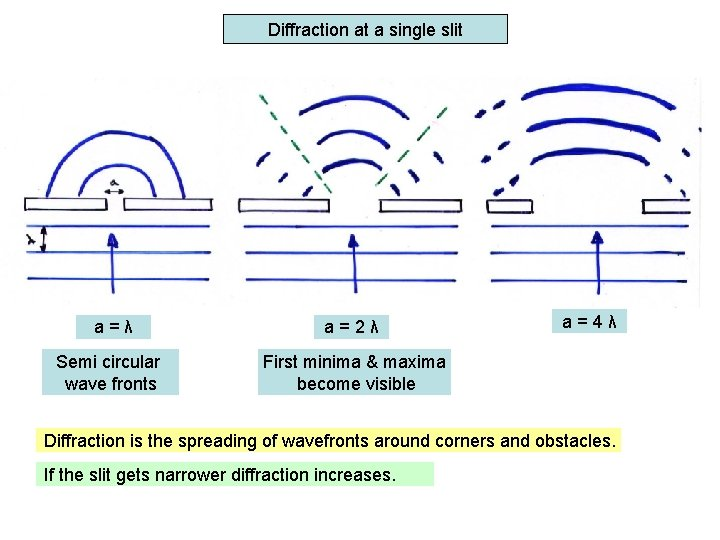Diffraction at a single slit a=λ a=2λ Semi circular wave fronts First minima & maxima become visible a=4λ Diffraction is the spreading of wavefronts around corners and obstacles. If the slit gets narrower diffraction increases. If the wavelength increases diffraction increases.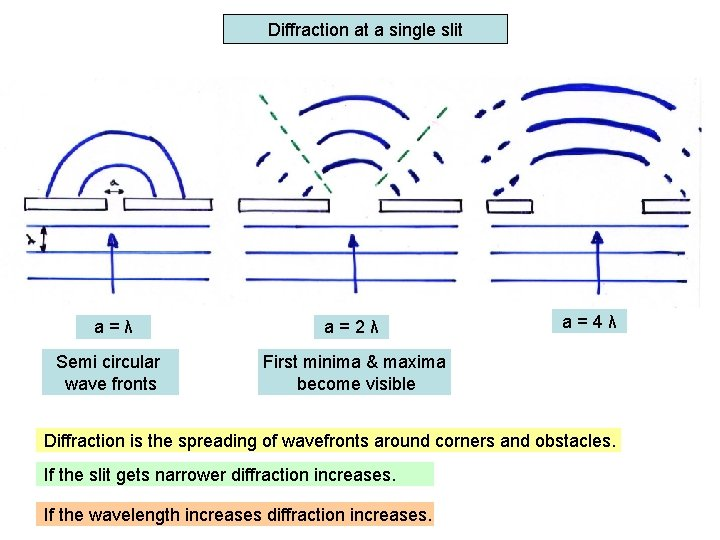Diffraction at a single slit a=λ a=2λ Semi circular wave fronts First minima & maxima become visible a=4λ Diffraction is the spreading of wavefronts around corners and obstacles. If the slit gets narrower diffraction increases. If the wavelength increases diffraction increases.It can be shown that the first minima occurs when sin Ə = λ/a. First minima T a Ə Central maxima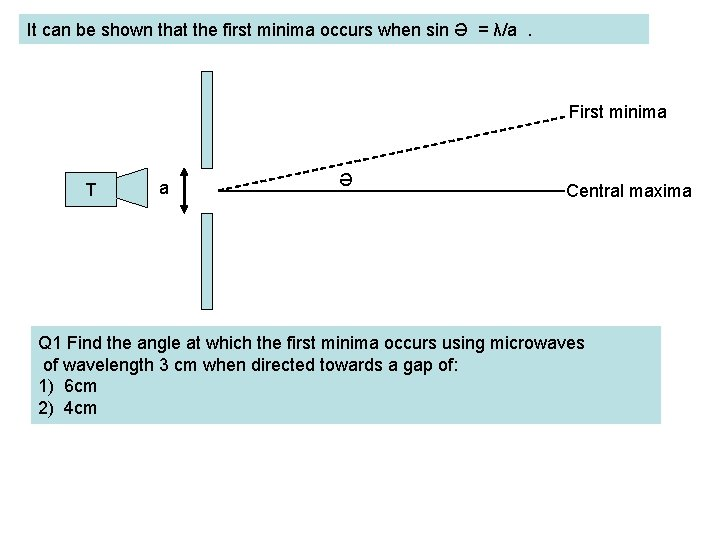It can be shown that the first minima occurs when sin Ə = λ/a. First minima T a Ə Central maxima Q 1 Find the angle at which the first minima occurs using microwaves of wavelength 3 cm when directed towards a gap of: 1) 6 cm 2) 4 cm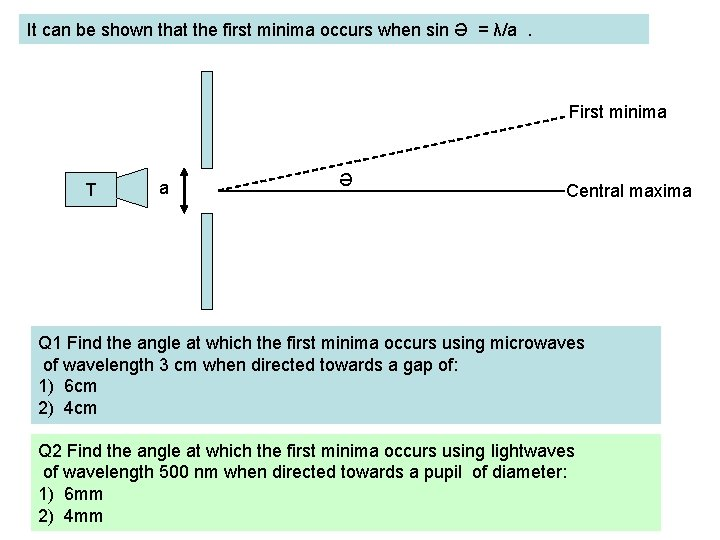It can be shown that the first minima occurs when sin Ə = λ/a. First minima T a Ə Central maxima Q 1 Find the angle at which the first minima occurs using microwaves of wavelength 3 cm when directed towards a gap of: 1) 6 cm 2) 4 cm Q 2 Find the angle at which the first minima occurs using lightwaves of wavelength 500 nm when directed towards a pupil of diameter: 1) 6 mm 2) 4 mm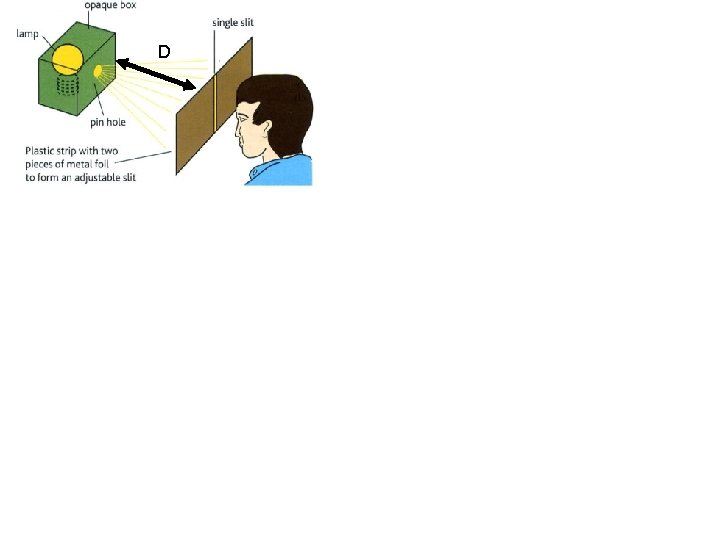DD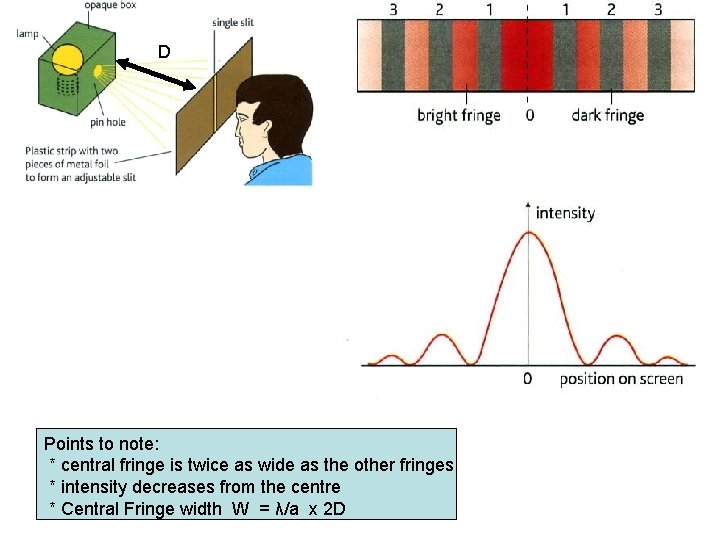D Points to note: * central fringe is twice as wide as the other fringes * intensity decreases from the centre * Central Fringe width W = λ/a x 2 DD Visit : http: //www. phys. hawaii. edu/~teb/optics/java/slitdiffr/ Points to note: * central fringe is twice as wide as the other fringes * intensity decreases from the centre * Central Fringe width W = λ/a x 2 D Blue light has narrower fringes So cameras and microscopes can see more detail using blue filters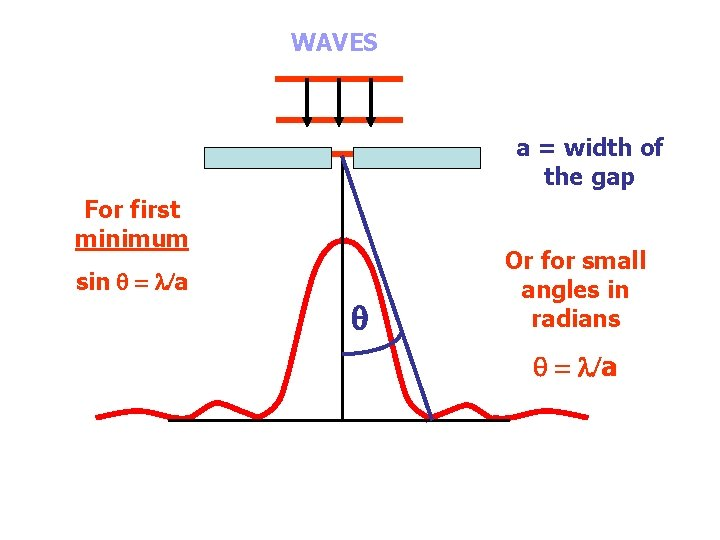WAVES a = width of the gap For first minimum sin = l/a Or for small angles in radians = l/aHow do you get the minima Q A a C B λ λ/2 D wave trains arrive in phase at the central maxima. Q First minima AQ is λ/2 longer than CQ so is out of phase by π giving destructive interference Corresponding points along AB which are λ/2 apart also cause destructive interference.How do you get the minima Q A a C B λ wave trains arrive in phase at the central maxima. Q First minima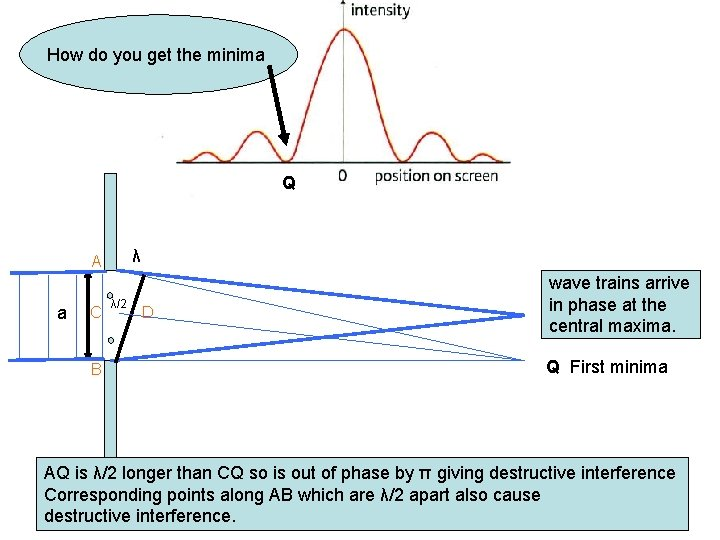How do you get the minima Q λ A a C B λ/2 D wave trains arrive in phase at the central maxima. Q First minima AQ is λ/2 longer than CQ so is out of phase by π giving destructive interference Corresponding points along AB which are λ/2 apart also cause destructive interference.How do you get the minima ? CD = λ = a sinƏ 2 2 λ = a sinƏ Q λ A a C B λ = sinƏ a λ/2 Ə D Q First minima AQ is λ/2 longer than CQ so is out of phase by π giving destructive interference Corresponding points along AB which are λ/2 apart also cause destructive interference.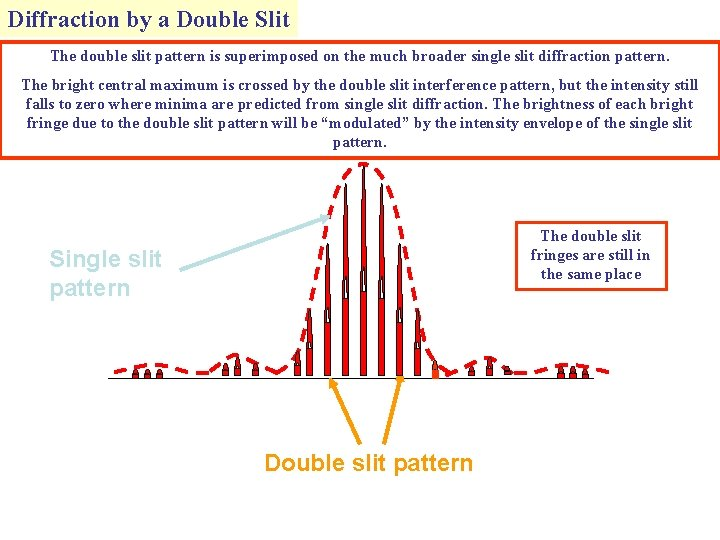Diffraction by a Double Slit The double slit pattern is superimposed on the much broader single slit diffraction pattern. The bright central maximum is crossed by the double slit interference pattern, but the intensity still falls to zero where minima are predicted from single slit diffraction. The brightness of each bright fringe due to the double slit pattern will be “modulated” by the intensity envelope of the single slit pattern. The double slit fringes are still in the same place Single slit pattern Double slit patternExperimental observations from the double slit i) For a pair of slits 0. 5 mm apart: λ red » λ blue ii) Using white light, fringes appear from all the various wavelengths present and do not overlap exactly, hence coloured fringes * Inner fringes are tinged with blue on the inside and red on Diffraction is the outside spreading of wavefronts around corners and obstacles. If the slit gets narrower diffraction increases. If the wavelength increases diffraction increases.Experimental observations from the double slit cont’d iii) Fringes obtained using slits 0. 5 mm apart drawn with different widths (a) (b) (a) thin slits Diffraction is the spreading of wavefronts around corners and obstacles. If the slit gets narrower diffraction increases. If the wavelength increases diffraction increases.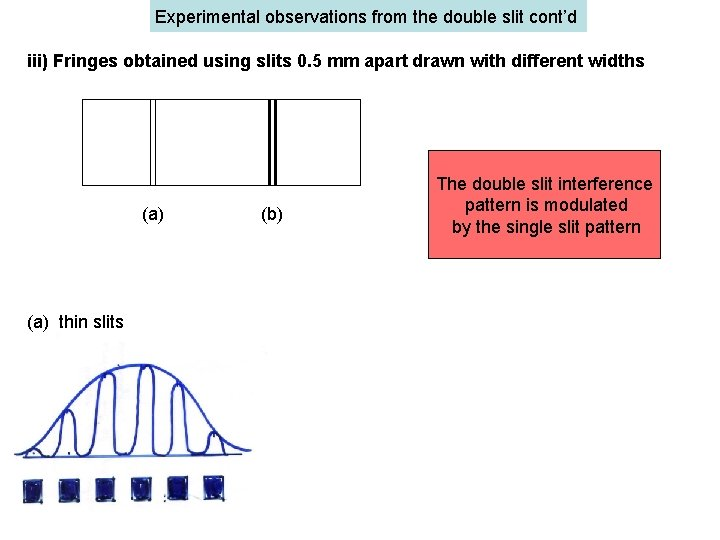Experimental observations from the double slit cont’d iii) Fringes obtained using slits 0. 5 mm apart drawn with different widths (a) (b) The double slit interference pattern is modulated by the single slit pattern (a) thin slits Diffraction is the spreading of wavefronts around corners and obstacles. If the slit gets narrower diffraction increases. If the wavelength increases diffraction increases.Experimental observations from the double slit cont’d iii) Fringes obtained using slits 0. 5 mm apart drawn with different widths (a) The double slit interference pattern is modulated by the single slit pattern (b) (a) thin slits (b) thick slits Missing fringes Diffraction is the spreading of wavefronts around corners and obstacles. If the slit gets narrower diffraction increases. If the wavelength increases diffraction increases.